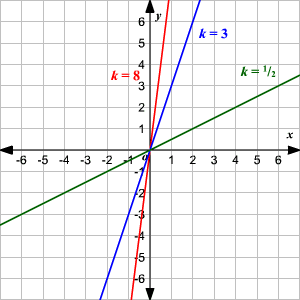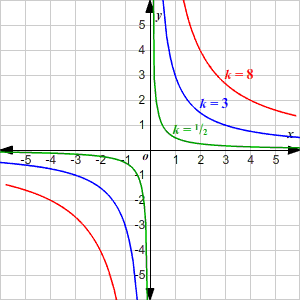# Direct & Inverse Variation

Direct variation describes a simple relationship between two variables . We say $y$ varies directly with $x$ (or as $x$ , in some textbooks) if:

$y=kx$

for some constant $k$ .

This means that as $x$ increases, $y$ increases and as $x$ decreases, $y$ decreases—and that the ratio between them always stays the same.

The graph of the direct variation equation is a straight line through the origin.Direct Variation Equation
for $3$ different values of $k$

Inverse variation describes another kind of relationship. We say $y$ varies inversely with $x$ (or as $x$ , in some textbooks) if :

$xy=k$ ,

or, equivalently,

$y=\frac{k}{x}$

for some constant $k$ .

This means that as $x$ increases, $y$ decreases and as $x$ decreases, $y$ increases.

The graph of the inverse variation equation is a hyperbola .Inverse Variation Equation
for $3$ different values of $k$